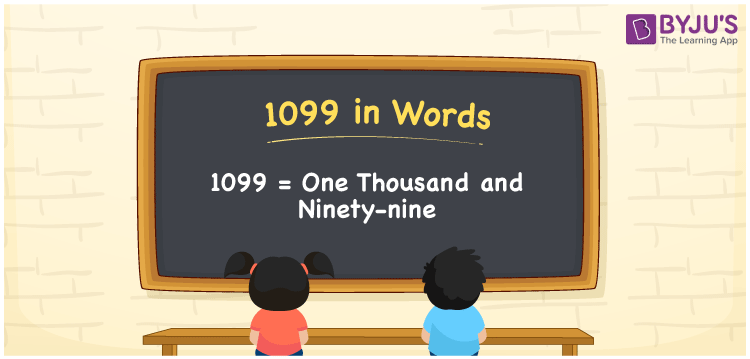# 1099 in Words

1099 in words is written as One thousand ninety-nine. In both the International System of Numerals and the Indian System of Numerals, 1099 is written as One thousand ninety-nine. The number 1099 is a Cardinal Number as it represents some quantity. For example, “the dress costs 1099 rupees”.

 1099 in Words One thousand ninety-nine One thousand ninety-nine in Number 1099

## 1099 in English Words

1099 in English words is read as “One thousand ninety-nine.”## How to Write 1099 in Words?

To write 1099 in words, we shall use the place value chart. In the place value chart, put 1 in the thousands, 0 in the hundreds, and 9 in the tens and the ones place respectively. Let us make a place value chart to write the number 1099 in words.

 Thousands Hundreds Tens Ones 1 0 9 9

Thus, we can write the expanded form as

1 × Thousand + 0 × Hundred + 9 × Ten + 9 × One

= 1 × 1000 + 0 × 100 + 9 × 10 + 9 × 1

= 1000 + 0 + 90 + 9

= 1099

= One thousand ninety-nine.

1099 is the natural number that is succeeded by 1098 and preceded by 1100.

1099 in words – One thousand ninety-nine

• Is 1099 an odd number? – Yes
• Is 1099 an even number? – No
• Is 1099 a perfect square number? – No
• Is 1099 a perfect cube number? – No
• Is 1099 a prime number? – No
• Is 1099 a composite number? – Yes

## Frequently Asked Questions on 1099 in Words

Q1

### How to write 1099 in words?

1099 in words is written as One thousand ninety-nine.
Q2

### How to write 1099 in words in the International and Indian System of Numerals?

In both, the system of numerals, 1099 in words, is written as One thousand ninety-nine.
Q3

### How to write 1099 in a place value chart?

In the place value chart, write 1 in the thousands, 0 in the hundreds, and 9 in the tens and the ones, respectively.